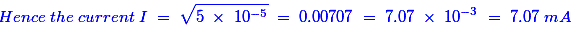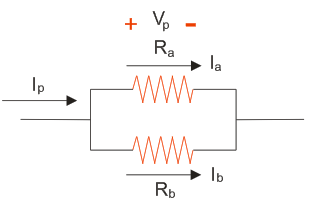# MCQs on Electric Circuits

##### Page 29 of 63. Go to page 1 2 3 4 5 6 7 8 9 10 11 12 13 14 15 16 17 18 19 20 21 22 23 24 25 26 27 28 29 30 31 32 33 34 35 36 37 38 39 40 41 42 43 44 45 46 47 48 49 50 51 52 53 54 55 56 57 58 59 60 61 62 63
01․ You have to replace 1500 Ω resistor in radio. You have no 1500 Ω resistor but have several 1000 Ω ones which you would connect
two in parallel.
two in parallel and one in series.
three in series.
three in parallel.

If we connect two 1000 Ω resistors in parallel, then the total resistance is the 500 Ω. Now, if we connect this parallel combination of two 1000 Ω resistance in series with another 1000 Ω resistance, then the total resistance is 1500 Ω. Hence, we need three 1000 Ω resistors with the connection of two in parallel and one in series.

02․ The electrical resistance of human body is around
5 ohms.
25 ohms.
250 ohms.
1000 ohms.

The electrical resistance of the human body is how much electricity a human body can handle. The electrical resistance of the human wet body is 1,000 ohms.

03․ The maximum current rating for a 10 k Ω, 0.5 W resistor is
0.707 mA.
7.07 mA.
14.14 mA.
28.28 mA.

We know that the expression of Power P = I2R. Now, I2 = P / R = 0.5 / 10000= 5.0 Ω 10-5.04․ When current flows through heater coil it glows but supply wiring does not glow because
supply wiring is covered with insulation layer.
current through supply line flows at slower speed.
resistance of heater coil is more than the supply wires.
supply wires are made of superior materials.

Heater coil is made up of alloys such as Manganin wire, and it is having high melting point. Also it has high resistance. Due to the high resistance, more heat will be generated. Hence, it will glow. But, the heater wire does not glow because it is having less resistance than the heater coil.

05․ Two resistor are said to be connected in parallel when
both carry the same value of current.
total current equals the sum of branch currents.
both have the same potential difference.
both ‘b’ and ‘c’

If we connect two resistors in parallel, then the voltage is same across the two resistors and the total current divides into two paths. The current flow through the two parallel connected resistors is shown in below figure.06․ When resistance element of a heater fuses and then we reconnect it after removing a portion of it, the power of heater will
decrease.
increase.
remain constant.
none of these.

If we remove a portion of resistance in that initial resistance, then the current resistance value will be decreased. This leads to flow more current than the previous case. Hence, according to the power equation (P = VI), if current increases then the power of the heater also increases.

07․ Which of following materials has the least resistivity ?
Zinc.
Copper.
Mercury.

It is a transition metal on the periodic table (atomic number 29) with a reddish- brown color body. It resists corrosion and is a good conductor and has a high conductivity when it comes to electricity. Hence, it has the least resistivity.

08․ Which resistor will be physically larger in size?
100 Ω, 10 W.
10 Ω, 50 W.
1 M Ω, 1/2 W.
1 k Ω, 1 W.

The power consumption of 10 Ω, 50 W resistor is high. If the power consumption is high, then the physical dimensions are also high.

09․ Three identical resistors are first connected in parallel and then in series. The resultant resistance of the first combination to the second will be
9 times.
1 / 9 times.
3 times.
1 / 3 times.

If we connect three identical resistors, R in parallel, then the equivalent resistance is [R / 3]. If we add that three [R] resistances in series, then the equivalent resistance is [3R]. Now the resultant resistance of the first combination to the second will be [R / 3] / 3R = [1 / 9].

10․ A resistance having rating 10 ohms, 10 W is likely to be a
metalic resistor.
carbon resistor.
wire wound resistor.
variable resistor.

The total current flowing through this resistance is 1 Amp and it is very low resistance. It has very high power rating. The wire wound resistors can be produced very accurate, and have excellent properties for low resistance values and high power ratings. Because the wire wound resistors are made up of an alloy such as nickel-chromium (nichrome) or a copper-nickel-manganese alloy called Manganin. This is having very good capability to dissipate the heat which is produced by the current flowing through the material (resistor).

<<<2728293031>>>